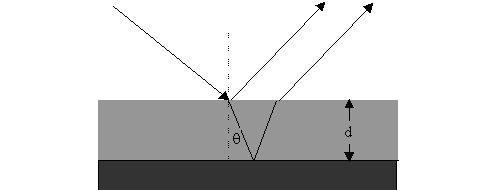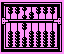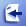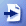Section 2.9: Reflection and Absorption of Laser Beams

If the surface being machined reflects too much light energy, the absorbed energy is decreased, the operation efficiency is lowered, and the reflected light may do harm to the optical systems. So reflection and absorption of laser beams is closely related to laser machining.

The value of absorption and reflection is related by:

Reflectivity = 1- Absorptivity (for opaque materials) or

Reflectivity = 1- Absorptivity - Transmissivity (for transparent materials)Figure2.19: Light transmission in medium

The reflection coefficient R for normal angles of incidence from air to opaque perfect flat clean metal surface can be computed using the following formula:

R=[(1-n)2+k2]/[(1+n)2+k2]

and the absorptivity A of opaque metal surface is:

A= 1 – R =4n/[(n+1)2+k2]

Where n is the refraction coefficient of material, k is the extinction coefficient of material. Both value can be looked up in handbooks. We list some of the values in the following table.Remember these optical properties are functions of radiation wavelength and varies with temperature.

Table 2.1: Some material's optical properties (under 1.06 micro radiation)

 Materials k n R Al 8.50 1.75 0.91 Cu 6.93 0.15 0.99 Fe 4.44 3.81 0.64 Mo 3.55 3.83 0.57 Ni 5.26 2.62 0.74 Pb 5.40 1.41 0.84 Ti 4.0 3.8 0.63 W 3.52 3.04 0.58 Zn 3.48 2.88 0.58 Sn 1.60 4.70 0.46 Glass 0 1.5 0.04

Next we examine the factors that affect reflectivity and absorptivity.

Wavelength: the shorter the wavelength, the more energetic the photons are. Photons with shorter wavelengths are easier to be absorbed by the materials than photons with longer wavelengths. Thus R normally decreases as wavelength becomes shorter, while absorption increases when photon energy increases.

Temperature: as temperature rises, there will be an increase in the phonon population. Electrons are more likely to interact with the structure rather than with the incident photons. So there is a fall in the reflectivity and an increase in the absorptivity with a rise in temperature.

Angle of Incidence and Plane of Polarization: reflectivity varies with both the angle of incidence and plane of polarization. If the plane of polarization is in the plane of incidence, the ray is called parallel ray ("p" ray); if the plane of polarization is perpendicular to the plane of incidence, the ray is called "s" ray. The reflectivity coefficients for perfect flat surfaces of the "p" ray and "s" ray are:

Rp=[(n-1/cosf )2+k2] / [(n+1/cosf )2+k2]

Rs=[(n-cosf )2+k2] / [(n+cosf )2+k2]

Where f is the incident angle, n is the refraction coefficient, k is the material extinction coefficient. We see here the reflectivities of p ray and s ray are different, p rays are more easily absorbed by materials than s rays do. You can try the following animation to see the difference.

G2.9 (Left): How Rp changes with n, f and k; (Right): How Rs changes with n, f and k

You can change the incident angle to see the reflection changes. k=6.93 for Cu.Example: Using data in table 2.3, find the s-ray and p-ray reflection and absortion of Nd:YAG laser beam radiation on Al surfaces, the incident angle is 60 degree.

Solution: For Al, k=8.5, n=1.75,

Rs = [(n-cosf )2+k2] / [(n+cosf )2+k2] = [(1.75-cos60)^2+8.5^2]/[(1.75+cos60)^2+8.5^2] = 73.8125 / 77.3125 = 0.955 =95.5%

Absorption for s-ray = 1-Rs = 4.5%

Rp = [(n-1/cosf )2+k2] / [(n+1/cosf )2+k2] = [(1.75-1/cos60)^2+8.5^2]/[(1.75+1/cos60)^2+8.5^2] = 72.3125 / 86.3125 = 83.8%

Absorption for p-ray = 1- Rp =16.2%Example: Using data in table 2.3, find the s-ray and p-ray reflection and absortion of Nd:YAG laser beam radiation on Fe surfaces, the incident angle is 60 degree.

Solution: For Fe, k=4.44, n=3.81,

Rs = [(n-cosf )2+k2] / [(n+cosf )2+k2] = [(4.44-cos60)^2+3.81^2]/[(4.44+cos60)^2+3.81^2] = 77.18%

Absorption for s-ray = 1-Rs = 22.82%

Rp = [(n-1/cosf )2+k2] / [(n+1/cosf )2+k2] = [(4.44-1/cos60)^2+3.81^2]/[(4.44+1/cos60)^2+3.81^2] = 36.56%

Absorption for p-ray = 1- Rp =63.44%

Comment: 1) For the same laser beam, different materials have very different absortion coefficient;

2) For the same laser beam, s-ray and p-ray absortion for same material can also be very different.